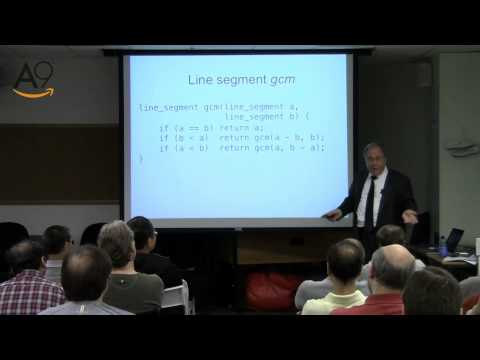A9 Videos► Play all

Four Algorithmic Journeys Part 2: Heirs of Pythagoras

• A9 Videos
• 12 videos
• 3,887 views
• Last updated on Oct 31, 2012
The Second Journey covers Euclid's GCD algorithm and its relation to diverse problems, such as sequence rotation.

Topics * Pythagoras. Universality of numbers. Discovery of irrational quantities.
* Euclid, Elements and Euclidean algorithm.
* Egyptian algorithm for division with remainder. Archimedes and his axiom.
* Remainder gcd.
* Simon Stevin and polynomials. Polynomial rings. Polynomial division with remainder. Polynomial gcd.
* Generalization of Euclidean algorithm: Euclidean rings. Emmy Noether and the birth of abstract algebra.
* Groups, Cosets, Lagrange Theorem.
* Rings, Ideals.
* Extended Euclidean algorithm. Multiplicative inverse algorithm.
* Stein algorithm. Stein algorithm for polynomials. Group of units in an integral domain. Smallest prime in an integral domain. Generalizing Stein algorithm.
* Permutation group. Cycle decomposition. Rotations of a sequence. The cyclic structure of a rotation. Rotation algorithms.
The Second Journey covers Euclid's GCD algorithm and its relation to diverse problems, such as sequence rotation.

Topics * Pythagoras. Universality of numbers. Discovery of irrational quantities.
* Euclid, Elements and Euclidean a...